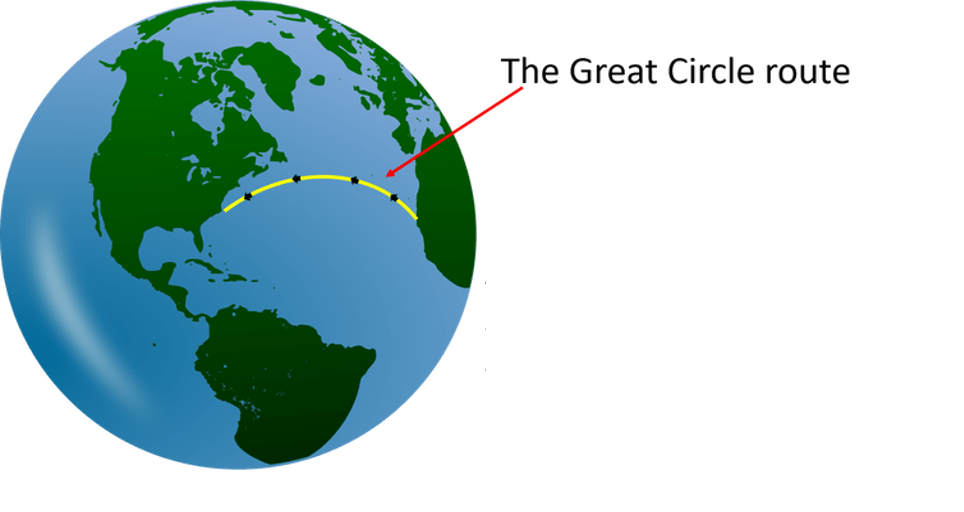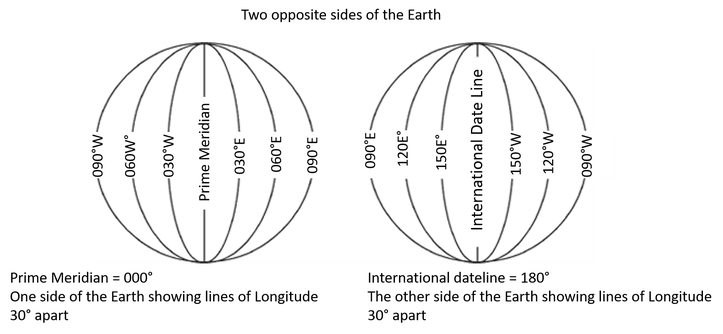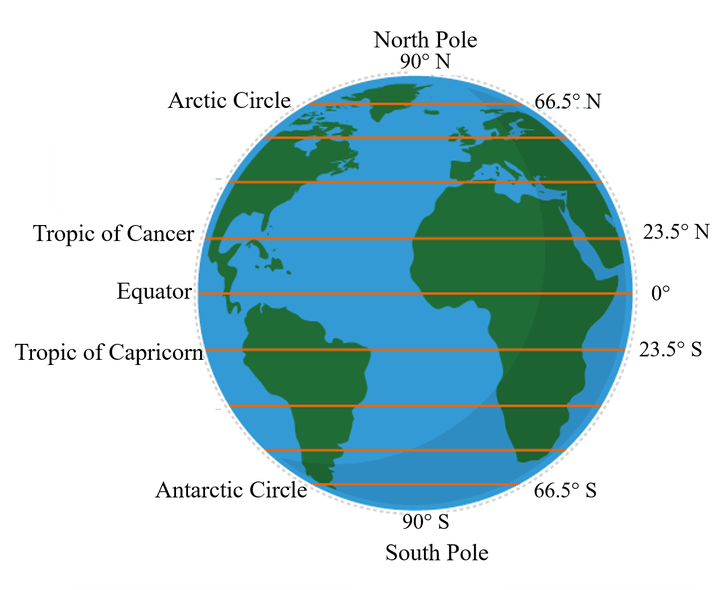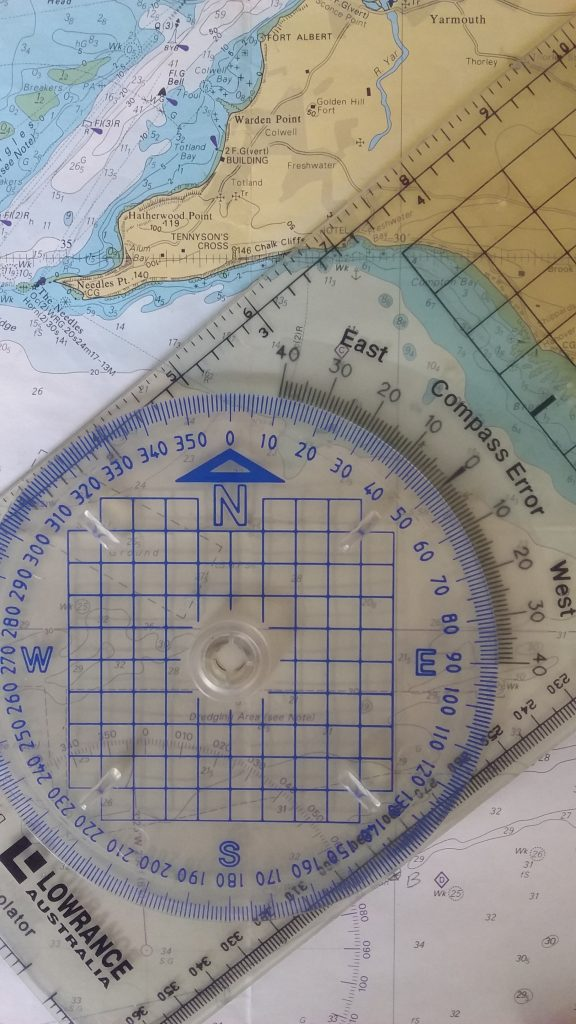# Articles

## Double the Angle off your Bow

Finding your position with double the angle off the bow This calculation takes advantage of the properties of an isosceles triangle. An isosceles triangle has two sides of equal length and consequently, it has two internal angles that are also equal. This rule is used when the second bearing between the bow of your boat … Continue reading Double the Angle off your Bow

## DistanceNautical Mile/One Minute of Latitude We know that we measure in degrees (°), minutes (’) and seconds (ʺ)  or decimals of a minute. One minute of latitude is equal to one nautical mile (1,852 metres or 1.852 kilometres). TIP: You MUST measure distance from the latitude scale adjacent to the area you are working in … Continue reading Distance

## Degrees, Minutes, and Seconds – Decimalise Example

Conversions If you are calculating the time you will arrive at your destination, you would use the Speed, Distance, Time formula. To enable the use of a calculator we must convert the minutes (or seconds as per the example) to a decimal. Once calculated, the navigator would not say, "We will arrive in 3.5 hours, … Continue reading Degrees, Minutes, and Seconds – Decimalise Example

## Latitude and Longitude

Writing latitude and longitude correctly is extremely important. The next person on watch must be able to read and understand what you have written on the chart and in the log book. Latitude should always be written first (in some areas in the USA longitude is written first, latitude first is more widely accepted): E.g.                         … Continue reading Latitude and Longitude

## The Great CircleThe Great Circle is the largest circle that can be drawn around the Earth’s sphere. When cutting a sphere exactly in half you have cut along one of its great circles. All Meridians are great circles. The Equator is a great circle (i.e. cutting the Earth in half). Great circles are useful in planning routes … Continue reading The Great Circle

## How are Longitude Angles Measured?The green shaded area depicts the angle measured from the centre of the Earth to the Prime Meridian to the longitude line west (in this example, measured at 080°W) B is the centre of the Earth C is the Prime Meridian A is the 080°W line of longitude The orange shaded area depicts the angle … Continue reading How are Longitude Angles Measured?

## Longitude (Meridians)The imaginary line drawn from the North Pole extending to the South Pole and running through Greenwich is the Prime Meridian. Just as the Equator divides the north and south hemispheres, the Prime Meridian divides the globe into east and west hemispheres. All lines on the Earth’s surface which run north to south as per … Continue reading Longitude (Meridians)

## How are Latitude Angles Measured?Latitude is measured by the angle at the Earth’s centre between the place in question and the equator. This image shows: The equator is at 0° The latitude of the North Pole is 90°N The latitude of the South Pole is 90°S The latitude of every point between must be either: Measured in degrees north … Continue reading How are Latitude Angles Measured?

## LatitudeWhat do the Co-ordinates Mean? To find an exact position on Earth we must have two coordinates: Latitude and Longitude. Latitude and longitude are the lines that are drawn on charts (and maps). It is standard practice (and easier) to decimalise the minutes in order to use formulas for navigation. Latitude The equator: An imaginary … Continue reading Latitude

## Where on Earth are we?More importantly, what does that mean? Navigation is basically the art of determining where you are and what direction and distance you must go. Therefore, we must learn to find out where we are and what that means for us. We do this by understanding the nautical chart, plotting our position, and laying off direction … Continue reading Where on Earth are we?